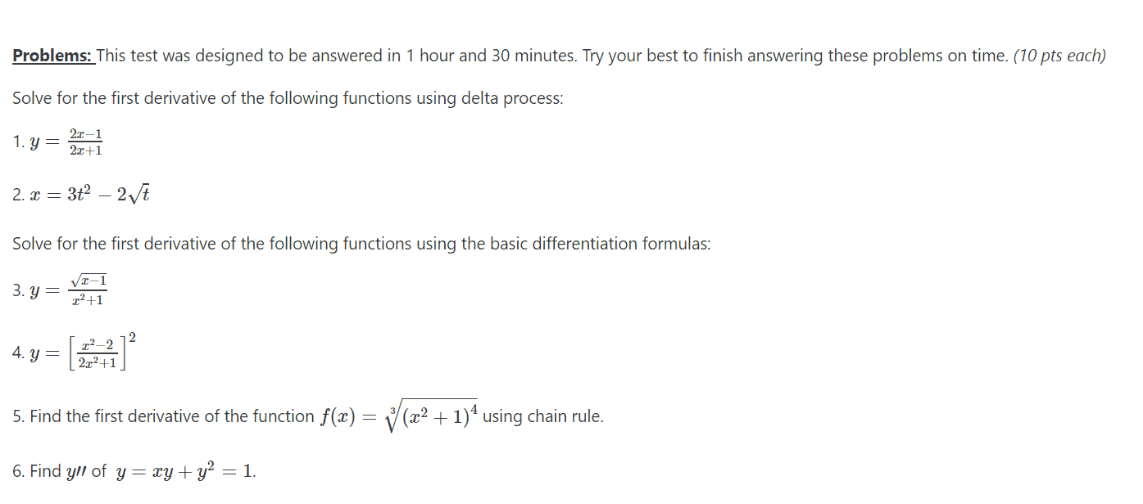# Derivatives quiz delta process differentiation formulas and techniques of differentiation

• MHB
Gwapakoskeeter
uhh ... is this a "test" for us to do to see if we can beat the set time limit?

If it is for you to complete, have you made any attempt on any of these six problems?

btw, looks like #6 has a typo ... one of those two equal signs is maybe a plus sign?

HOI
The first two problems ask you to find the derivative using the "delta process". Do you know what that is?

The next two problem asks you to find the derivative "using the basic differentiation formulas". Do you know what those are?

The fifth problem asks you to find the derivative "using the chain rule". Do you know what that is?